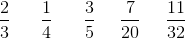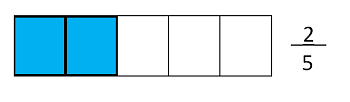What is Fractional Notation? - Definition & Conversion Video

An error occurred trying to load this video.

Try refreshing the page, or contact customer support.

Coming up next: What is a Hexadecimal? - Definition & Conversion

You're on a roll. Keep up the good work!

Replay
Your next lesson will play in 10 seconds
• 0:04 Choices
• 0:31 What is Fractional Notation?
• 1:10 Visualizing Fractional…
• 1:39 Mixed Numbers
• 2:18 Conversion
• 3:40 Lesson Summary
Save Save

Want to watch this again later?

Timeline
Autoplay
Autoplay
Speed Speed

Recommended Lessons and Courses for You

Lesson Transcript
Instructor: Nola Bridgens

Nola has taught elementary school and tutored for four years. She has a bachelor's degree in Elementary Education, a master's degree in Marketing, and is a certified teacher.

In this lesson, we will learn what fractional notation is and what it looks like. We will also learn how to convert mixed numbers to fractional notation.

Choices

If you had a choice between eating 1 1/3 of a candy bar or 4/3 of a candy bar, which would you choose? Did you know that a mixed number (like 1 1/3) and an improper fraction (like 4/3) can represent the exact same amount? These two numbers are equal, so no matter which one you chose, you would have the same amount of candy bar! This lesson will show you why this is true and how to convert mixed numbers to improper fractions.

What Is Fractional Notation?

We use fractions when we are referring to a part of a whole instead of a whole number. Fractional notation simply means that a number is written in fraction form. It is written as a/b where neither a nor b is equal to 0.

A fraction has two parts, the numerator and denominator. The numerator is the number on top of the line and it represents how many pieces of the whole we are referring to. The denominator is the number below the line, and it represents how many pieces make up one whole. Look at these examples:Visualizing Fractional Notation

It's said that a picture is worth a thousand words, so let's look at some images that will help us understand fractional notation. The following image shows a bar broken into 5 equal pieces. Therefore, 5 pieces make up a whole bar in this example, so the denominator is 5. Of the 5 pieces, 2 are shaded in. Because we are referring to 2 of the 5 pieces, the numerator in this example is 2. This image represents the fraction 2/5.Mixed Numbers in Fractional Notation

We can also write mixed numbers in fractional notation. A mixed number is one that consists of a whole number and a fraction. When we convert a mixed number to fractional notation, it makes an improper fraction. An improper fraction is a fraction that represents more than one whole.

To unlock this lesson you must be a Study.com Member.

Register to view this lesson

Are you a student or a teacher?

See for yourself why 30 million people use Study.com

Become a Study.com member and start learning now.
Back
What teachers are saying about Study.com

Earning College Credit

Did you know… We have over 200 college courses that prepare you to earn credit by exam that is accepted by over 1,500 colleges and universities. You can test out of the first two years of college and save thousands off your degree. Anyone can earn credit-by-exam regardless of age or education level.